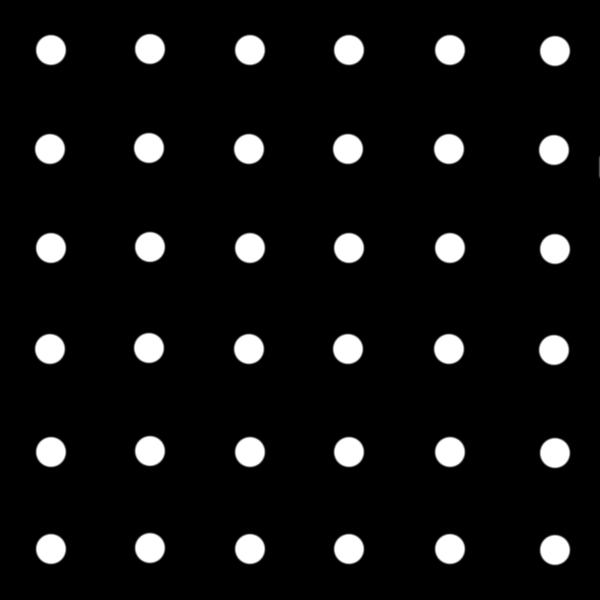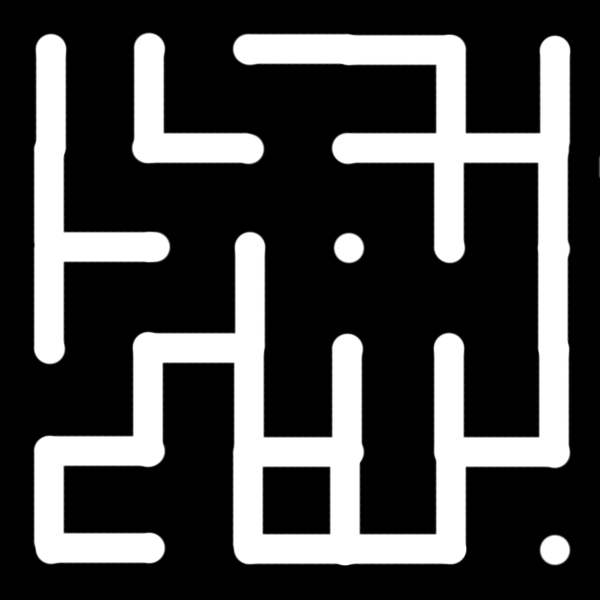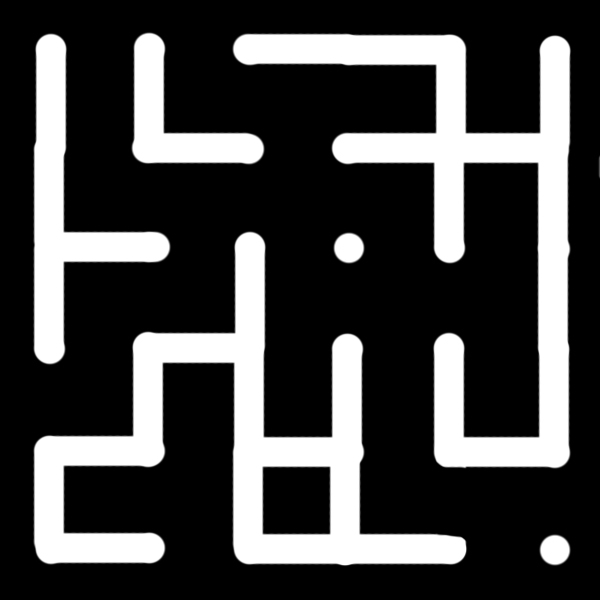## The Random Maze Puzzle

Frequent readers of my blog will remember a few weeks ago (or a few months or years depending on when you are reading this) that I presented an interesting mathematical puzzle called The Random Maze. It is such a beautiful and yet complex problem that I am almost certain someone has thought of it before, but as yet neither I nor the mathematicians that I have corresponded with have been able to find a reference to it before my own work. (And if someone can provide a citation for if and when it was first proposed, please contact me and let me know)Suppose that you have an N x M lattice of points, where N and M are two integers, such as the one depicted above. For every pair of horizontal and vertical neighbouring points (ie everything except diagonal) randomly decide whether to connect the points or not. You can use a coin toss, or dice, or any random number generator. When you are finished, you should have a randomly generated maze, such as the ones below.As you can see in these two examples, the one on the right can be solved, while the one on the left cannot be. (I am assuming that a solution must start in the top left and end in the bottom right). The question is, given the probability that a specific pair of neighbouring points are connected, what is the probability that the entire maze can be solved?

## Hints:

Among the people I have shown this puzzle to, including several mathematicians, no one has been able to find a general solution to it. It seems like the sort of puzzle that should have a simple solution, but spending a few hours working on it shows that it also has many complications. Based on some of the comments and replies I received from my original article, as well as my own work on the puzzle, here are a few hints regarding the solution:

• By symmetry properties, it is reasonable to assume that when p = 1/2, then the total probability of solving the maze is also P(1/2) = 1/2. It is also reasonable to assume that P (p) = P ( 1 - p) for the same reasons. (Which you should be able to work out for yourselves :) )
• Many of the proposed solutions I have received accidentally assumed that a maze can only be solved with paths that always travel down or to the right. These probabilities are less than the true solution, because it is possible for a maze to be solvable even when the solution goes all the way to the bottom left, and then rises to the top right before coming back down to the end point.
• Some people also assumed that it was possible to have solutions that leave the maze and then return to it later, in that they allowed N or M to go to infinity.
• A very common error that I have seen is assuming that the maze changes while being solved. For example, if my solution goes right one unit and then left one unit, that has a probability of p, because once I know that path is clear it remains clear. Some people thought it had a probability of p^2 because there is a probability p that you can go right, and a probability p that you can go back left.
• And while this may seem trivial since a solution of a maze should not travel through the same part of the path twice, it does have implications when calculating the probability that a single maze will have two or three or more solutions. You cannot assume that the probability of a maze having two solutions is the square of the probability of having a single solution, because they are highly correlated. Surprisingly this one critical point makes the solution of this puzzle a lot harder!
• For the more advanced readers, this puzzle is closely related to problems in both the Ising Model and the 1-state Potts Model and the corresponding crossing functions (I intend to post a review of the Q-state Potts model (and more details on its application to the maze problem) on my science blog when I get more time). And while the crossing functions can be calculated for certain values of p, and the solution to this puzzle can be written in terms of crossing functions, I contend that the solution of this problem can be calculated in a more general and less complicated manner than the Potts model crossing functions. But as always, I could be wrong.
• For those who want more of a challenge, I have also listed some interesting variations on this problem on another page.

So I will leave it to you the reader to play around with this puzzle and see if you can find the solution. It is a fun way to waste a few hours!Make a free website with Yola